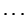Next: Converting old ratings to Up: Details of the Rating Previous: Standard rating formula

## Rating floors

The absolute rating floor for all ratings is 100. No rating can be lower than the absolute rating floor.

A player with an established rating has a rating floor possibly higher than the absolute floor. Rating floors exist at 100, 1400, 1500, 1600,, 2100. A player's rating floor is calculated by subtracting 200 points from the highest attained established rating, and then using the floor just below. For example, if an established player's highest rating was 1941, then subtracting 200 yields 1741, and the floor just below is 1700. Thus the player's rating cannot go below 1700. If an established player's highest rating was 1588, then subtracting 200 yields 1388, and the next lowest floor is 100, which is this player's floor.

Mark Glickman
2004-09-22